# ISEE Upper Level Quantitative : Variables and Exponents

## Example Questions

← Previous 1 3 4 5 6

### Example Question #1 : How To Add Exponential Variables

Simplify: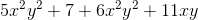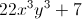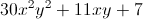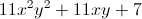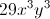The expression cannot be simplified furtherExplanation:

Group and combine like terms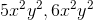: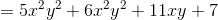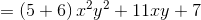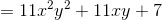### Example Question #2 : Variables And Exponents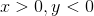Which is the greater quantity?

(a)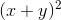(b)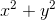(b) is greater.

(a) and (b) are equal.

(a) is greater.

It is impossible to tell from the information given.

(b) is greater.

Explanation: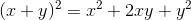Sinceandhave different signs,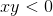, and, subsequently,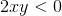Therefore,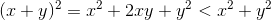This makes (b) the greater quantity.

### Example Question #3 : Variables And Exponents

Assume thatandare not both zero. Which is the greater quantity?

(a)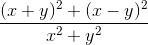(b)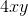It is impossible to tell from the information given.

(a) and (b) are equal.

(b) is greater.

(a) is greater.

It is impossible to tell from the information given.

Explanation:

Simplify the expression in (a):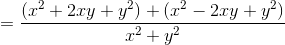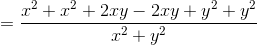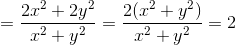Therefore, whether (a) or (b) is greater depends on the values ofand, neither of which are known.

### Example Question #4 : Variables And Exponents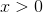Which is the greater quantity?

(a)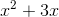(b)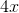(a) and (b) are equal

(b) is greater

It is impossible to tell from the information given

(a) is greater

It is impossible to tell from the information given

Explanation:

We give at least one positive value offor which (a) is greater and at least one positive value offor which (b) is greater.

Case 1:(a)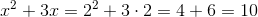(b)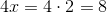Case 2: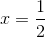(a)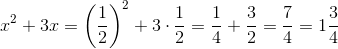(b)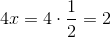Therefore, either (a) or (b) can be greater.

### Example Question #1 : How To Add Exponential Variables

Assume all variables to be nonzero.

Simplify: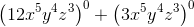None of the answer choices are correct.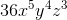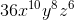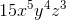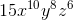None of the answer choices are correct.

Explanation:

Any nonzero expression raised to the power of 0 is equal to 1. Therefore,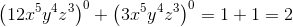.

None of the given expressions are correct.

### Example Question #6 : Variables And Exponents

Simplify: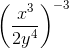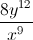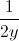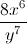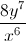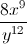Explanation: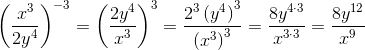### Example Question #7 : Variables And Exponents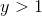Which is greater?

(a)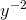(b)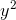(a) and (b) are equal

It is impossible to tell from the information given

(a) is greater

(b) is greater

(b) is greater

Explanation:

If, then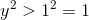and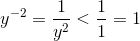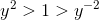, so by transitivity,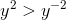, and (b) is greater

### Example Question #1 : How To Find The Exponent Of Variables

Expand: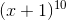Which is the greater quantity?

(a) The coefficient of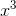(b) The coefficient of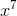The two quantities are equal.

(a) is greater.

It is impossible to tell from the information given.

(b) is greater.

The two quantities are equal.

Explanation:

By the Binomial Theorem, if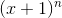is expanded, the coefficient ofis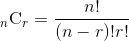.

(a) Substitute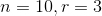: The coerfficient ofis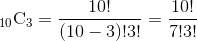.

(b) Substitute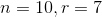: The coerfficient ofis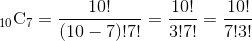.

The two are equal.

### Example Question #61 : Variables

Which is greater?

(a)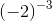(b)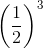(b) is greater.

It is impossible to tell from the information given.

(a) and (b) are equal.

(a) is greater.

(b) is greater.

Explanation: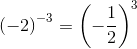A negative number to an odd power is negative, so the expression in (a) is negative. The expression in (b) is positive since the base is positive. (b) is greater.

### Example Question #2 : How To Find The Exponent Of VariablesWhich is the greater quantity?

(a)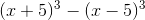(b)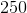(b) is greater.

It is impossble to tell from the information given.

(a) is greater.

(a) and (b) are equal.

(a) is greater.

Explanation:

Simplify the expression in (a):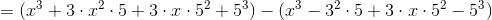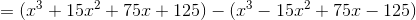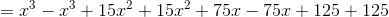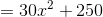Since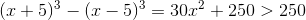,

making (a) greater.

← Previous 1 3 4 5 6